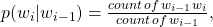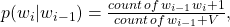# Given a bigram language model, in what scenarios do we encounter zero probabilities? How Should we handle these situations ?

1. Recall the Bi-gram model can be expressed as :
2.1. Scenario 1 – Out of vocabulary(OOV) words – such words may not be present during training and hence any probability term involving OOV words will be 0.0 leading entire term to be zero.
1. This is solved by replacing OOV words by UNK tokens in both training and test set and adding UNK to the vocabulary.
2. Scenario 2 – Not all bi-grams(n-grams in case of n-gram language model) exist in training set but might be present in the test set. For ex., If the entire corpus is “This is the only sentence in the corpus”, and you need to find the probability of a sequence like “this is the sentence in the corpus”,p(sentence | the) = 0.0 as bi-gram “the sentence” doesn’t occur in the training set, but the test sequence is highly probable given the training set.
1. This is solved by smoothing techniques such as adding a constant in numerator and denominator both, such that probabilities don’t nullify but are very small in default.
2. For ex., Laplacian smoothing is add-k smoothing where k >= 1
3. Instead oftakewhereis the vocabulary size.